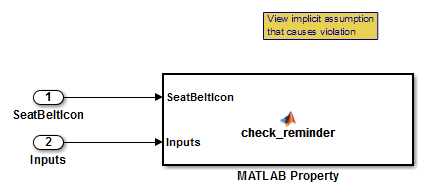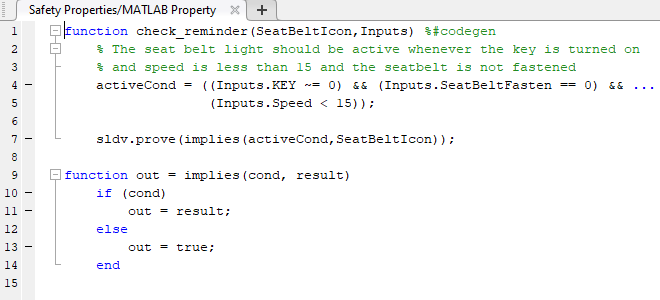sldv.prove

Proof objective function for Stateflow charts and MATLAB Function blocks

Description

example

sldv.prove(expr) specifies that expr is true for every evaluation while proving properties. Use any valid Boolean expression for expr.

This function has no output and no impact on its parenting function, other than any indirect side effects of evaluating expr. If you issue this function from the MATLAB® command line, the function has no effect.

Intersperse sldv.prove proof assumptions within the code or separate the assumptions into a verification script.

Examples

collapse all

Specify a property proof objective and proof assumption in sldvdemo_sqrt_blockrep model by using a MATLAB Function block.

Open the sldvdemo_sbr_verification model and save it as ex_sldvdemo_sbr_verification.

Open the Safety Properties subsystem.Open the MATLAB Property block, which is a MATLAB Function block.At the end of the check_reminder function definition, add sldv.assume(Inputs.KEY==0 | 1); so that the last two lines of the function definition are:

sldv.prove(implies(activeCond, SeatBeltIcon));
sldv.assume(Inputs.KEY==0 | 1);

To save the updated code, in the Editor tab, click Save and close the editor.

To prove the safety properties, in the Simulink® Editor, select the Safety Properties subsystem. On the Design Verifier tab, click .

Alternatively, in the Simulink Editor, you can right-click the Safety Properties subsystem and select Design Verifier > Prove Subsystem Properties.

Input Arguments

collapse all

MATLAB expression, for example, x > 0.

Alternatives

Instead of using the sldv.prove function, you can insert a Proof Objective block in your model. Using sldv.prove instead of a Proof Objective block offers several benefits, described in What Is Property Proving?.

You can also specify a proof objective by using MATLAB for code generation without using the sldv.prove function. Using sldv.prove instead of directly using MATLAB for code generation eliminates the need to:

• Express the objective by using a Simulink block.

• Explicitly connect the proof output to a Simulink block.